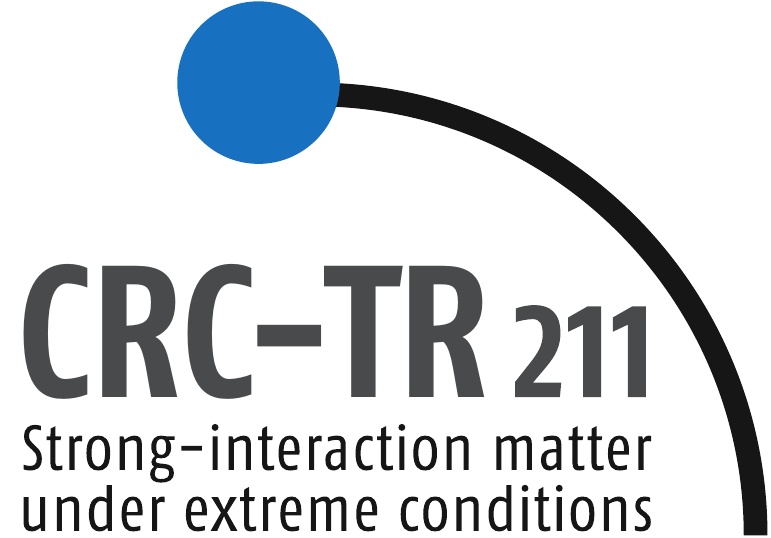#Transport Meeting

Venue: Institute for Theoretical Physics, Goethe University Frankfurt, Physics Building, PHYS 2.114
Time: Thursday, June 06, 2:15pm
Contact: hees@th.physik.uni-frankfurt.de

# Non-equlibrium photons from the thermalizing Glasma

## Oscar Garcia (Heidelberg University)

Recently, a parametric estimate of the total yield of non-equilibrium photons was derived in the  BMSS thermalization scenario. It was found that the total yield was comparable to the thermal contribution, specially at higher values of the saturation scale $Q_S^2$. In this work, we expand on this estimates, by calculating the $p_\perp$ resolved spectra for the BMSS three stages by parametrizing the findings of far-from-equilibrium classical statistical lattice simulations. These scaling solutions are used in a small angle kinetic rate to calculate the photon spectra for the thermalizing matter, where analytical solutions were found. We find also the asymptotics used in the previous work for the total yield. We constrain our system using charge hadron multiplicities from LHC and RHIC as in previous work on these parametric estimates.

Nuclear Physics Colloquium Homepage